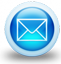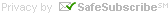# Construction Calculator

Concrete Calculator: This is the simple construction calculator will help an estimator to decide how many yards of concrete slab he needs to pour for roofing. This yardage calculator has these options to fill by the estimators:

• Length
• Width
• Depth
• The estimator will get the button of Calculate The Yard
• The estimator will get the button of Calculate Another Area - to vacate the old calculated data.

Paint Calculator: This calculation will help you determine how many gallons of paint you will need to paint a wall, or a room.

When you are calculating paint:

• Calculate the area you will be painting by using this simple calculator.
• Dont forget to subtract the windows and doors.
• A door opening = 20 Sqft.
• A window opening = 15 Sqft.
• Usually you can expect (1) gallon of paint to Cover 350 sqft of an area. However, this will Take more paint if the area is un-prepared, or if you are changing colors.

Masonry Calculator: This calculator will ask you the height and the length specifically.

Use this calculator to figure out how many 8x8x16 cmu units you will need to build a wall.

CMU Wall Unit Calculator

Insert all dimensions on feet

This calculates units of 8"x 16" CMU block

Sheetrock Calculator: This calculationr will help you determine how many sheets of 4x8 sheets of gypsum board you will need to cover an area.

Insert all dimensions on feet:

• This calculates how many sheets of drywall you need to cover an area.
• When you are calculating Sheetrock
• Calculate the area you will be covering by using this simple calculator. But don't forget to subtract the windows and doors.
• A door opening = 20 Sqft.
• A window opening = 15 Sqft. This calculator is based on the use of 4'0"x8'0" sheets of sheetrock.The formula used is W*L/32.

How to Estimate Concrete needed for a Job:

How to transpose drawing:

How to splice Rebar:# Takeoff Tools